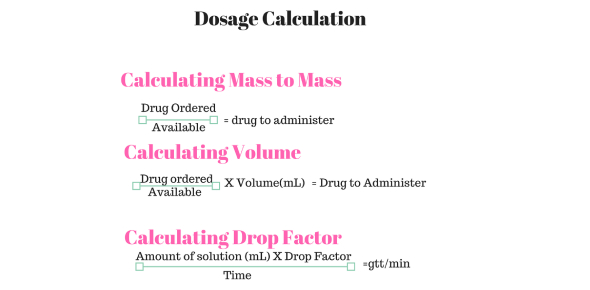# Drug Dosage Calculations NCLEX Quiz 6

10 Questions | Total Attempts: 1691SettingsAll questions are shown, but the results will only be given after you’ve finished the quiz. You are given 1 minute per question, a total of 10 minutes in this quiz.

• 1.
Keflin 2 g in 100 ml D5W IVPB over 20 minutes. The I.V. tubing is 15 gtt/ml. Calculate the flow rate.
• A.

150 gtt/min

• B.

100 gtt/min

• C.

75 gtt/min

• D.

76 gtt/min

• 2.
The physician writes an order for Heparin 900 units/hour. The label on the I.V. bag reads: Heparin 10.000 units in 500 ml D5W. How many ml/hr will deliver the correct dose?
• A.

11 ml/hr

• B.

45 ml/hr

• C.

50 ml/hr

• D.

55 ml/hr

• 3.
Administer Heparin 1.000 units/hr from an I.V. bag mixed 40.000 units in 1 L D5W. How many ml/hr will deliver the correct amount of Heparin?
• A.

40 ml/hr

• B.

30 ml/hr

• C.

25 ml/hr

• D.

15 ml/hr

• 4.
The patient’s Heparin is infusing at 28 ml/hr on an infusion pump. The bag of fluid is mixed 20.000 units of Heparin in 500 ml D5W. What hourly dose of heparin is the patient receiving?
• A.

1.120 units/hr

• B.

1.210 units/hr

• C.

2.120 units/hr

• D.

2.210 units/hr

• 5.
The patient’s heparin drip is infusing at 11 ml/hr on an infusion pump. The bag of fluid is mixed 25.000 units of Heparin in 250 ml D5W. What hourly dose of Heparin is the patient receiving?
• A.

2.500 units/hr

• B.

2.000 units/hr

• C.

1.000 units/hr

• D.

1.100 units/hr

• 6.
Give Regular insulin by continuous I.V. infusion at 20 units/hr. The solution is 250 ml NS with 100 units of Regular insulin. What rate on the infusion pump will deliver the correct dose?
• A.

5 ml/hr

• B.

8 ml/hr

• C.

50 ml/hr

• D.

100 ml/hr

• 7.
Administer a Theophylline drip at 40 mg/hr The solution is 250 ml D5W + Theophylline 500 mg. What rate on the infusion pump will deliver the correct dose?
• A.

20 ml/hr

• B.

40 ml/hr

• C.

80 ml/hr

• D.

100 ml/hr

• 8.
Give Tridil 15 mcg/min. Tridil is mixed 50 mg in 500 ml D5W. What rate on the infusion pump will deliver the correct dose?
• A.

150 ml/hr

• B.

15 ml/hr

• C.

90 ml/hr

• D.

9 ml/hr

• 9.
Give propofol 10 mcg/kg/minute. The infusion is mixed propofol 250 mg in 250 ml D5W. The patient weighs 168 pounds. What rate on the infusion pump will deliver the correct dose?
• A.

10 ml/hr

• B.

26 ml/hr

• C.

46 ml/hr

• D.

50 ml/hr

• 10.
Give Nitroprusside 5 mcg/kg/minute via continuous infusion for a patient weighing 205 lbs. Nitroprusside is available in a solution of 200 mg in 250 ml D5W. What rate on the infusion pump will deliver the correct dose?
• A.

25 ml/hr

• B.

35 ml/hr

• C.

45 ml/hr

• D.

50 ml/hr

Related TopicsBack to top